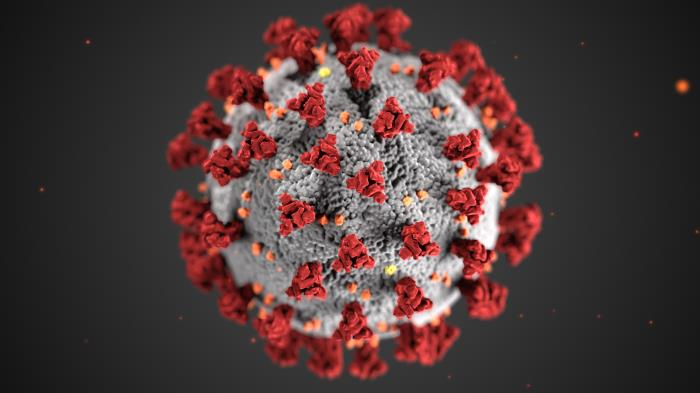Analysis of live COVID-19 data – a MATLAB/Octave approach

March 25, 2020Analysis of (time-series) data being collected in (near) realtime often provides insight for a process underway. As the current situation with COVID-19 is still critical lots of data are being collected, curated and streamed throughout the world, and researchers strive to get the most out of them to identify patterns and, maybe, make predictions. In this post I am showing how to download and analyse live data using MATLAB/Octave.

First thing is to find the data sources, which should in any case be reliable. For the purposes of this post I am going to use two sources:

Then, those data should be downloaded and parsed to be useful for processing and visualisation. In the scenario adopted in this post, what is of interest is to create a view of the confirmed cases and deaths normalised by the country population, for a selection of countries, in this case China, Italy, Spain, United Kingdom, United States of America, Greece.

Let’s take a look at the code that downloads and processes the data. Suppose we are considering the data from GitHub.

sourceURL = 'https://open-covid-19.github.io/data/data.csv';
theDataFormat = '%D%s%s%s%s%f%f%f%f%f';
data = string2table( live_data, theHeaderFormat, theDataFormat);
data.CountryCode = categorical( data.CountryCode);
data.CountryName = categorical( data.CountryName);
data.RegionCode = categorical( data.RegionCode);
data.RegionName = categorical( data.RegionName);
confirmed_cases_column = 5;
deaths_column = 6;
population_column = 9;
data = table2timetable( data );

The conversion of some fields into categorical is not mandatory at this point. The auxiliary function string2table is transforming the string containing all the downloaded data into a meaningful table as shown in the following piece of code. The X_column variables denote the columns in the data table that contain the corresponding X data. The table2timetable just converts the table to a time series table.

function tabl = string2table ( s, headfmt, datafmt )
Fields = cellfun(@(x) x{1}, textscan(s, headfmt, 1, 'Delimiter', ','), 'un', 0);
theData = textscan(s, datafmt, 'Headerlines', 1, 'EndOfLine', newline, 'Delimiter', ',');
tabl = table(theData{:}, 'VariableNames', Fields);

Then all we need to do is define the countries of interest and go ahead with the plots.

countries_of_interest = {'China','Italy','Spain','United Kingdom','United States of America','Greece'};

Suppose we want to create graphs, one for the normalised confirmed cases and one for the normalised reported deaths. The normalisation is supposed to be based on the respective country population, so that the graph actually corresponds to the power the country loses. First, let’s create the confirmed cases graph.

figure; hold on; set(gcf,'position',[1000 1000 800 500])
for c = 1:length(countries_of_interest)
% read the confirmed cases column from the data
country_data = data(find(data.CountryName==countries_of_interest{c}), confirmed_cases_column);
% read the population column from the data
country_population = data(find(data.CountryName==countries_of_interest{c}), population_column);
% select the population reported in the first occurrence of a country
population = country_population.Population(1);
% plot the normalised cases as a percentage
stem(country_data.Date, 100*country_data.Confirmed/population);
end
% make it a bit nicer
title( 'Normalised confirmed cases - source: GitHub');
legend( countries_of_interest, 'location','northwest');
xlabel( 'Time'); ylabel( '% of total country population');
set( gca, 'Fontsize', 16);
axis tight; grid on; box;
hold off;

This code will create a graph that will look like the following figure.

In pretty much the same way let’s create the normalised deaths graph.

figure; hold on; set(gcf,'position',[1000 1000 800 500])
for c = 1:length(countries_of_interest)
% read the deaths column from the data
country_data = data(find(data.CountryName==countries_of_interest{c}), deaths_column);
% Read the population column from the data
country_population = data(find(data.CountryName==countries_of_interest{c}), population_column);
% select the population reported in the first occurrence of a country
population = country_population.Population(1);
% plot the normalised deaths as a percentage
stem(country_data.Date, 100*country_data.Deaths/population);
end
title( 'Normalised deaths - source: GitHub');
legend( countries_of_interest, 'location','northwest');
xlabel( 'Time'); ylabel( '% of total country population');
set( gca, 'Fontsize', 16);
axis tight; grid on; box;
hold off;

This code will create a graph that will look like the following figure.

Following the same strategy, bellow are the respective graphs created by using the data provided by data.world (which may look a bit different from the previous graphs due to either more or less data).